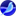Author Topic: gattMath is a math program that show some concepts behind Calculus  (Read 1893 times)

0 Members and 1 Guest are viewing this topic.

Software Santa

•• Posts: 4447
• OS:
•Mac OS X 10.9
• Browser:
•SeaMonkey 2.30gattMath is a math program that show some concepts behind Calculus
« on: November 10, 2014, 11:54:41 PM »
gattMath is a free educational math program that show some concepts behind Integral and Differential Calculus

Quote
Description

gattMath is a educational math (graphical) program that show some concepts behind Integral and Differential Calculus, like Riemann, Simpson, Darboux, Trapezoid, Monte-Carlo integration, derivative, antiderivative, tangent,..., and sure a plotter.

Categories
Education, Mathematics

GNU General Public License version 2.0 (GPLv2)

Features

2D and 3D Plotter
Riemann left, right, middle and random sum
Trapezoidal and Simpson's rule
Darboux sum
Monte-Carlo integration method
Derivative

gattMath
is a free educational math program that show some concepts behind Integral and Differential Calculus, like Riemann, Simpson, Darboux, Trapezoid and Monte-Carlo sum, derivative, tangent, ... and sure a plotter (2D and 3D).
The intention is to help students from universities or any introductory Calculus courses, illustrating some points of the Integral and Differential Calculus based on Numeric Calculus.

The main of the program is the integration algorithms (at least, showing their geometric (graphical) meaning with of course the correspondent result.

Supported interface languages: English, Portuguese, French.

 gattMath 1.5 ZIPWindows XP, Vista, Seven gattMath 1.5 ZIPLinux, Mac, Solaris, others...: need Java Virtual Machine (JVM 6+)

http://gattmath.sourceforge.net/calculus/index.php

http://sourceforge.net/projects/gattmath/

This Site was Opened on January 1st, 2007

Welcome Visitor:

 @ MEMBER OF PROJECT HONEY POTSpam Harvester Protection Networkprovided by Unspam

email